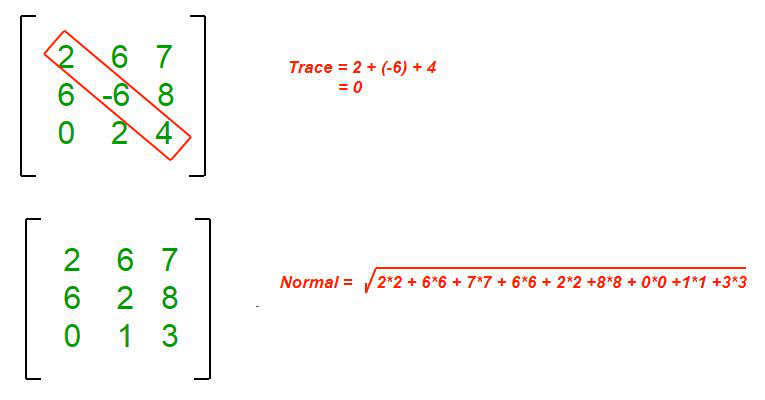Open In App

# C Program To Find Normal and Trace of Matrix

Here, we will see how to write a C program to find the normal and trace of a matrix. Below are the examples:

Input: mat[][] = {{1, 2, 3},
{4, 5, 6},
{7, 8, 9}};
Output:

• Normal = 16
• Trace  = 15

Explanation:

• Normal = sqrt(1*1+ 2*2 + 3*3 + 4*4 + 5*5 + 6*6 + 7*7 + 8*8 + 9*9) = 16
• Trace  = 1+5+9 = 15

Input: mat[][] = {{5, 6, 1},
{7, 2, 9},
{6, 1, 3}};
Output:

• Normal = 10
• Trace  = 10

Explanation:

• Normal = sqrt(5*5+ 6*6 + 1*1 + 7*7 + 2*2 + 9*9 + 6*6 + 1*1 + 3*3) = 15
• Trace  = 5+2+3 = 10

For a better understanding see the below image.Approach:

1. To Find Normal:

• Run nested loop to access elements of the matrix.
• Find the sum of all the elements present in the matrix.
• Then return the square root of that sum.

2. To Find Trace:

• Run a single loop to access diagonal elements of the matrix.
• Return the sum of diagonal elements.

Below is the C program to find normal and the trace of a matrix:

## C

 `// C program to find trace ``// and normal of given matrix``#include ``#include `` ` `// Returns Normal of a matrix ``// of size n x n``int` `findNormal(``int` `mat[], ``               ``int` `n)``{``    ``int` `sum = 0;``   ` `    ``// Run nested loop to access ``    ``// elements of matrix``    ``for` `(``int` `i = 0; i < n; i++)``        ``for` `(``int` `j = 0; j < n; j++)``            ``sum += mat[i][j] * mat[i][j];``    ``return` `sqrt``(sum);``}`` ` `// Returns trace of a matrix of ``// size n x n``int` `findTrace(``int` `mat[], ``int` `n)``{``    ``int` `sum = 0;``   ` `    ``// Run a loop to access diagonal ``    ``// elements of matrix``    ``for` `(``int` `i = 0; i < n; i++)``        ``sum += mat[i][i];``    ``return` `sum;``}`` ` `// Driven code``int` `main()``{``    ``int` `mat = {{1, 2, 3}, ``                     ``{4, 5, 6}, ``                     ``{7, 8, 9}};``    ``printf``(``"Normal of Matrix = %d"``, ``            ``findNormal(mat, 3));``    ``printf``(``"\nTrace of Matrix = %d"``, ``            ``findTrace(mat, 3));``    ``return` `0;``}`

Output

```Normal of Matrix = 16
Trace of Matrix = 15```

Time Complexity: O(n*n)
Space Complexity: O(1)